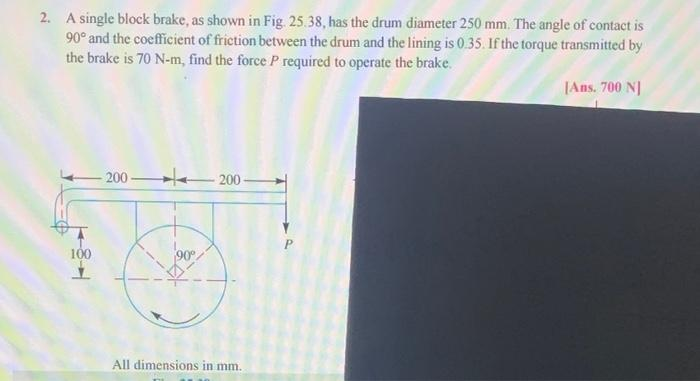# (Solved): machine design please step by step solution  2. A single block brake, as shown in Fig. 25. ...

machine design2. A single block brake, as shown in Fig. 25.38, has the drum diameter $$250 \mathrm{~mm}$$. The angle of contact is $$90^{\circ}$$ and the coefficient of friction between the drum and the lining is $$0.35$$. If the torque transmitted by the brake is $$70 \mathrm{~N}-\mathrm{m}$$, find the force $$P$$ required to operate the brake. |Ans. $$700 \mathrm{~N}$$ | All dimensions in $$\mathrm{mm}$$.